Questions If you are a professional pilot or your work involves professional aviation please use this forum for questions. Enthusiasts, please use the 'Spectators Balcony' forum.

# IAS to TAS formula?

Join Date: Apr 2008
Location: Brisbane
Age: 35
Posts: 61
IAS to TAS formula?

Is there any formula to get TAS from a IAS with varying temp or press?Join Date: May 2000
Location: Seattle
Posts: 3,150
Quick formula:

TAS = IAS * [1 + (Altitude/1000 * .02)]

Altitude in feet

Or, TAS = IAS + 2% per 1000' altitude.Join Date: Aug 2000
Location: California
Posts: 1,196
TAS=IAS/sqrt(delta)

where Delta=ratio of air density to ISA SL density
=288.15/(T+273.15) * (P/1013.25)

and P= Ambient pressure in HPa(mB)
T= ambient temperature in degrees celsius

This ignores the compressibility correction, which is very small at low Mach numbers up to about M0.3

The "rule of thumb" correction is actually nearer to 1.5% per 1000' at low altitudesJoin Date: Aug 2003
Location: Sale, Australia
Age: 76
Posts: 3,828
From Aviation Formulary V1.43
Mach numbers, true vs calibrated airspeeds etc.

Mach Number (M) = TAS/CS
CS = sound speed= 38.967854*sqrt(T+273.15) where T is the OAT in celsius.
TAS is true airspeed in knots.

Because of compressibility, the measured IAT (indicated air temperature) is higher than the actual true OAT. Approximately:

IAT=OAT+K*TAS^2/7592

The recovery factor K, depends on installation, and is usually in the range 0.95 to 1.0, but can be as low as 0.7. Temperatures are Celsius, TAS in knots.

Also:

OAT = (IAT + 273.15) / (1 + 0.2*K*M^2) - 273.15

The airspeed indicator measures the differential pressure, DP, between the pitot tube and the static port, the resulting indicated airspeed (IAS), when corrected for calibration and installation error is called "calibrated airspeed" (CAS).

For low-speed (M<0.3) airplanes the true airspeed can be obtained from CAS and the density altitude, DA.

TAS = CAS*(rho_0/rho)^0.5=CAS/(1-6.8755856*10^-6 * DA)^2.127940 (DA<36,089.24ft)

Roughly, TAS increases by 1.5% per 1000ft.

When compressibility is taken into account, the calculation of the TAS is more elaborate:

DP=P_0*((1 + 0.2*(IAS/CS_0)^2)^3.5 -1)
M=(5*( (DP/P + 1)^(2/7) -1) )^0.5 (*)
TAS= M*CS

[(*) If this results in M>1 - ie supersonic flight, we have to account for the shock wave ahead of the pitot tube, using Rayliegh's Supersonic Pitot equation.

Using the M from above as the first guess on the RHS, iterate:

M=0.881285 sqrt((DP/P + 1)(1 - 1/(7*M^2))^(5/2))

to convergence.]

P_0 is is (standard) sea-level pressure, CS_0 is the speed of sound at sea-level, CS is the speed of sound at altitude, and P is the pressure at altitude.

These are given by earlier formulae:

P_0= 29.92126 "Hg = 1013.25 mB = 2116.2166 lbs/ft^2
P= P_0*(1-6.8755856*10^-6*PA)^5.2558797, pressure altitude, PA<36,089.24ft
CS= 38.967854*sqrt(T+273.15) where T is the (static/true) OAT in Celsius.
CS_0=38.967854*sqrt(15+273.15)=661.4786 knots

[Example: CAS=250 knots, PA=10000ft, IAT=2°C, recovery factor=0.8
DP=29.92126*((1+0.2*(250/661.4786)^2)^3.5 -1)= 3.1001 "
P=29.92126*(1-6.8755856*10^-6 *10000)^5.2558797= 20.577 "
M= (5*( (3.1001/20.577 +1)^(2/7) -1) )^0.5= 0.4523 Mach
OAT=(2+273.15)/(1 + 0.2*0.8*0.4523^2) - 273.15= -6.72C
CS= 38.967854*sqrt(-6.7+273.15)=636.08 knots
TAS=636.08*0.4523=287.7 knots]

In the reverse direction, given Mach number M and pressure altitude PA, we can find the IAS with:

x=(1-6.8755856e-6*PA)^5.2558797
ias=661.4786*(5*((1 + x*((1 + M^2/5)^3.5 - 1))^(2/7.) - 1))^0.5 (for M <=1)

Some notes on the origins of some of the "magic" number constants in the preceeding section:

6.8755856*10^-6 = T'/T_0, where T' is the standard temperature lapse rate and T_0 is the standard sea-level temperature.

5.2558797 = Mg/RT', where M is the (average) molecular weight of air, g is the acceleration of gravity and R is the gas constant.

0.2233609 = ratio of the pressure at the tropopause to sea-level pressure.

4.806346*10^-5 = Mg/RT_tr, where T_tr is the temperature at the tropopause.

4.2558797 = Mg/RT' -1

0.2970756 = ratio of the density at the tropopause to the density at SL (rho_0)

145442 = T_0/T'

38.967854 = sqrt(gamma R/M) (in knots/Kelvin^0.5), where gamma is the ratio of the specific heats of airJoin Date: Mar 2000
Location: Arizona USA
Posts: 8,572
Hmmm, I usually just read the TAS on the appropriate FD gauge, or look at the FMS.
Works for me...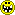Join Date: Apr 2008
Location: Brisbane
Age: 35
Posts: 61
wow, thanks for the response guys

Brian Abraham that looks like a really complex way of doing it, but im sure very accurate, but i would probably be at my destination before if figured it out.

I was aware of the TAS=IASx(alt/1000)x0.2 or 0.15......
BUT is there any correction you can make to for ISA temp deviation or ISA MSL press deviation??

thanksJoin Date: Aug 2003
Location: Sale, Australia
Age: 76
Posts: 3,828
brns2, sorry but you did ask
Is there any formula to get TAS from a IAS with varying temp or press?
Just didn't want you to say I left something out.I'm one of those nerds that wants the answer to the Nth decimal place.
411A, have yet to fly something with a TAS readout, has always been the whiz wheel.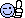The only Lockheed (12A) was so rudimentary it had a morse key in the RHS seat, quite unlike your chariot.Join Date: Aug 2000
Location: California
Posts: 1,196
If it helps:
Every 3 degrees Celsius above ISA approximates to a 1% decrease in delta and a 0.5% increase in TAS correctionJoin Date: May 2000
Location: Seattle
Posts: 3,150
IOW, if you're using the rule of thumb in the first place, it just doesn't matter!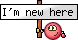Join Date: Sep 2017
Location: USA
Posts: 2
For low-speed (M<0.3) airplanes the true airspeed can be obtained from CAS and the density altitude, DA.

TAS = CAS*(rho_0/rho)^0.5=CAS/(1-6.8755856*10^-6 * DA)^2.127940 (DA<36,089.24ft)

Roughly, TAS increases by 1.5% per 1000ft.

When compressibility is taken into account, the calculation of the TAS is more elaborate:

DP=P_0*((1 + 0.2*(IAS/CS_0)^2)^3.5 -1)
M=(5*( (DP/P + 1)^(2/7) -1) )^0.5 (*)
TAS= M*CS
How do we type in this formula into an Excel spreadsheet?

DP=P_0*((1 + 0.2*(IAS/CS_0)^2)^3.5 -1)
M=(5*( (DP/P + 1)^(2/7) -1) )^0.5 (*)
TAS= M*CS

Thanks.Join Date: Mar 2014
Location: The World
Posts: 1,274
Hmmm, I usually just read the TAS on the appropriate FD gauge, or look at the FMS.
Works for me...Another example of "children of magenta"? Thou shall not use electronic equipment without being able to monitor its plausible working condition ...Moderator

Join Date: Apr 2001
Location: various places .....
Posts: 6,589
411A, who departed to another place some time ago ... would be the last person to whom one might assign that comment ..Join Date: Jun 2001
Location: OZ
Posts: 991
There's a free app for the Apple world.Join Date: Mar 2005
Location: N/A
Posts: 3,393
ChickenHouse, the late departed 411A knew more about the business than you are likely to learn. Flew the big pistons and was flying a 1011 till his end. Dad helped design the DC-3, and had Howard Hughes as a house guest.

chr2017, this may be of help, Chapter III.

http://www.dtic.mil/dtic/tr/fulltext/u2/a280006.pdf

Or else just use this.

TAS Calculator

411A left and right respectively.
Attached Images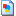b1.jpg (620.2 KB, 69 views)b4.jpg (412.9 KB, 66 views)Join Date: Oct 2004
Location: California
Posts: 302
Just use your E6B. It's right there on the calculator side. No electronics required.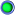Join Date: Jun 2001
Location: OZ
Posts: 991
Yeah right. The scale makes it very dodgy. The app is way better. OTOH you can get good results with the 12" model but it really won't fit in your pocket. Great for your ATP exams though.Join Date: Apr 2009
Location: On the Beach
Posts: 3,293
Every transport I flew had a TAS indicator. Even the "ancient" 707. Those ADCs do wonders.Join Date: Aug 2012
Location: N . Daarset
Age: 67
Posts: 189
1972 Whizz weel is still dng it business on a daily basis , and students seem to understand it .
Saving the planet with no electrics required .Join Date: Feb 2006
Location: UK
Age: 59
Posts: 20
Rule of thumb

I've always used TAS = IAS + IAS in miles per minute x altitude in 1000 ft - eg 300 kt IAS, 15 000 ft: TAS = 300 + (5 x 15) = 375 ktYou may not post new threads
You may not post replies
You may not post attachments
You may not edit your posts

BB code is On
Smilies are On
[IMG] code is On
HTML code is OffShow Printable VersionEmail this Page